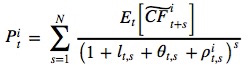### Why should I choose AnalystNotes?

AnalystNotes specializes in helping candidates pass. Period.

##### Subject 1. Framework for the Economic Analysis of Financial Markets
All financial assets represent a claim on the real economy. This reading explains the fundamental connection between the prices of financial assets and the underlying economy.

The Present Value Model

At any point in time, the market value of any financial security is simply the sum of discounted values of the cash flows that the security is expected to produce.where:

• Pit = the value of the asset I at time t (today)
• N = number of cash flows in the life of the asset
• CFit+s = the expectation of the random variable CF conditional on the information available to investors today (t)
• lt,s = yield to maturity on a real default-free investment today (t), which pays one unit of currency s periods in the future
• θ t,s = expected inflation rate between t and t+s
• pit,s = the risk premium required today to pay the investor for taking on risk in the cash flow of asset i, s periods in the future

The economy exerts its influences on the market value of financial instruments through the numerator and denominator.

The numerator is the asset's expected cash flows.

The denominator is the discount rate. It consists of three components.

• Real default-free interest rate. For investors preferring current to future consumption, it is the rate of compensation required to postpone current consumption. For example, the interest rate paid by T-bills is a risk-free rate of interest.

• Expected inflation rate. When prices are expected to increase, lenders charge not only an opportunity cost for postponing consumption but also an inflation premium that takes into account the expected increase in prices.

• Risk premiums. Companies exhibit varying degrees of uncertainty concerning their ability to repay lenders. Lenders therefore charge interest rates that incorporate default risk.

Therefore, the market value of an asset is affected by economic factors that affect the asset's expected future cash flows, default-free interest rates, expected inflation rates, and the asset's risk premium.

Expectations and Asset Values

Asset values depend on the expectations of future cash flows. These expectations are based on current information that may be relevant to forecasting future cash flows.

Information that changes expectations affects asset values.

• Anticipated information is already reflected in asset prices.
• Unanticipated or new information will change asset prices.